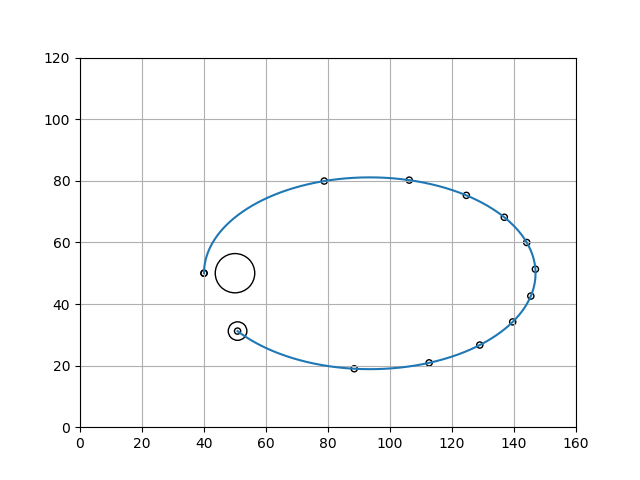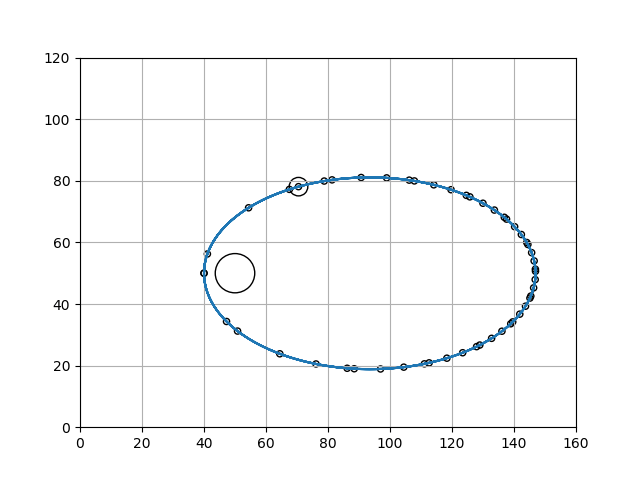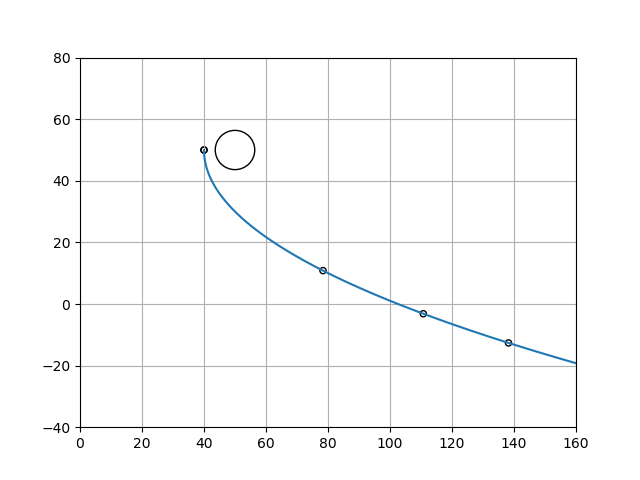# NS-Capture Simulation

The technology of the simulation is quite interesting and will be discussed on a separate page, however, this page will focus on describing the adaptation.

• The simulation is on hold until it is determined how to get javascript thru the WordPress framework.

The above simulation is in the process of being adapted to the NS-Capture Theory.

In the meantime, there is another approach that is also quite instructive. Rather than an animation, it consists of ellipse descriptions. For example, consider the following:Elliptical trajectory of a neutron star orbiting a regular giant star.

The diagram above shows the path of a neutron star in an elliptical orbit around a giant star. In reality, though, it is a diagram of a smaller mass orbiting a larger mass. The parameters of the object can be modified to represent stars or any other bodies, such as Earth around Sun, or Moon around Earth.

The program that draws the diagram is an amazing little piece of Python code that is available at:  https://astronomy.stackexchange.com/questions/7806/exercise-2d-orbital-mechanics-simulation-python

In the diagram above the dots along the curve are the position of the neutron star at equal time intervals. The time is measured by iterations through the main code loop, in this case, a dot is dropped every 10,000 iterations. One can tell the velocity of the neutron star by how much distance is between the dots. A longer distance means the neutron star has traveled further in the constant time interval, than shorted distances, which means it is going faster.

The NS is traveling fastest when it is closest to the star on the left of the diagram and slower when it is further from the NS on the right of the diagram.

The program is extremely accurate and can be used to determine velocity and position as a function of time around the ellipse. More on this accuracy will be discussed later, but it is mentioned now to give a sense of the remarkable capabilities of this program.

To show the accuracy, we can let the program run a little longer, so that it makes a 2nd traversal of the same elliptic orbit.The dot with the circle around it is the last dot in the sequence, so it can be seen the NS has made just over one and a half trips around the ellipse. Note that the dots from the first diagram above and that the new dots are interspersed. The new dots do not show up at the same location, because the full trip around the ellipse is not an exact multiple of the time interval at which the dots are dropped.

As indicated above, the highest velocity is when the NS is going around closest to the star (which is the larger circle in the left end of the ellipse. Note that the dot at (x,y) = (40,50) is left over from being the starting point of the first diagram, and that the dot at (50,30) was the last point on the first diagram (which had a circle around it). Also, the point at (80,80) was also in the first diagram.

Therefore the 2 points that represent the time interval around the left are the last point from the first diagram (50,30) and the point at roughly (68,78).

Also, the interval ((40,50)->(80,80)) from the first diagram is shorter than the interval ((50,30)->(68,78)) in the 2nd diagram, because the 2nd interval spends more time in the area of the stronger gravitational field and therefore has a higher velocity. The highest possible velocity for a time interval would be one with equal segments before and after the point of closest approach (40,50).

In terms of the accuracy of the program, one can get a good idea based on the points of the 2nd pass that clearly lie right on the ellipse of the first pass.

Let’s see how a couple more traversals of the ellipse looks:Note that the last point (circled) is still right on the ellipse line. Keep in mind that all these points (500,000 in this diagram, w 51 dots for the 10,000 iteration time segments) are calculated individually from the previous dot, along with the gravitational field at the previous dot. The technique used is called the “Euler Method”, for which there is relatively simple explanation here.

Getting back to the NS-Capture Theory, what happens is that a neutron star travelling through space has a chance encounter with an ordinary star. Their mutual gravitational fields interact and they are drawn together to the distance of closest approach (which varies depending on the initial positions and velocities of the NS and the regular star).

The following diagram shows the path of one such encounter with the NS entering from the right of the diagram and coming to the point of closest approach on the left at (50,40).If there was no other interaction than the gravitational fields and no distortions of the regular star in the presence of the neutron star, then the NS would simply fly away on a similar path on the top half of the diagram and never return.

However, the fact is that there will be an interaction and the regular star will be distorted, and as a result kinetic energy (1/2 m v**2) will be transferred to the regular star, which will slow down the NS to a smaller value of velocity, v’, at the distance of closest approach.

If the lower velocity, v’ < v, is less than the escape velocity, vEsc ( v’ < vEsc ), then the NS will be bound in an elliptical orbit similar to the first diagram above.

Then, unlike the 2nd diagram where the NS follows an identical path on the 2nd traversal, there will be a 2nd interaction, distorting the regular star and the velocity after passing the distance of closest approach, will again be less then the velocity from the previous pass, resulting in an ellipse that doesn’t reach as far to the right as it did on the first pass.

The distance will continue to decrease with each traversal until it eventually becomes circle, which, itself, will continue to tighten around the regular star until the neutron star is totally immersed within the atmosphere of the regular star.

It is the assertion of the NS-Capture theory that the presence of the NS in the regular star is what will eventually de-stabilize the regular star resulting in a supernova explosion.